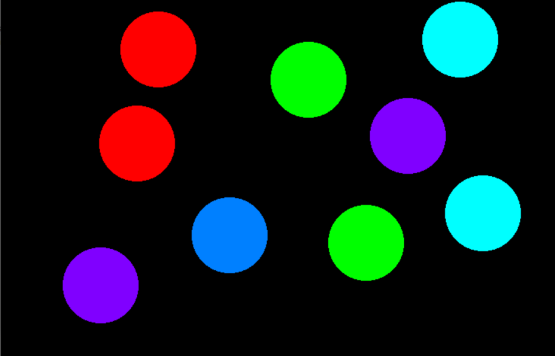GeeksforGeeks App
Open AppBrowser
Continue

# Program to draw circles using mouse moves in OpenGL

OpenGL: OpenGL is a cross-language, cross-platform API for rendering 2D and 3D Vector Graphics. It will make a lot of design as well as animations using this.

• Create a circle anywhere on the console using a single left mouse click and the coordinates of the center of the circle created depends on the position of your click.
• To change the color of the circle, simply right-click on the mouse.
• After performing all operations jump out of the program by simply pressing the Esc key on the keyboard.

Approach: The idea is to use the below inbuilt function to draw the circle using single click in OpenGL:

• glMatrixMode(GL_PROJECTION): This function sets the current matrix to projection.
• glLoadIdentity(): The function is used to multiply the current matrix by identity matrix.
• gluOrtho2D(0.0, 800.0, 0.0, 600.0): It sets the parallel(orthographic) projection of the full frame buffer.
• glutCreateWindow(“Circle Creation on mouse click”): Creates the window as specified by the user as above.
• glClearColor(0, 0, 0, 0): It sets the background color.
• glClear(GL_COLOR_BUFFER_BIT): It clears the frame buffer and set values defined in glClearColor() function call.
• glutDisplayFunc(display): It links the display event with the display event handler(display).
• glutMouseFunc(mouse): Mouse event handler.
• glutKeyboardFunc(keyboard): Keyboard event handler.
• glutMainLoop(): This function loops the current event.

Below is a C++ program that implements onClick functionality in OpenGL:

## C++

 `// C++ program to implement onClick``// functionality in OpenGL to draw``// a circle``#include ``#include ``#include ``#include ``#define xpix 500``#include ``using` `namespace` `std;` `float` `r, g, b, x, y;``bool` `flag = ``true``;``int` `counter = 0;` `// Function works on mouse click``void` `mouse(``int` `button, ``int` `state,``           ``int` `mousex, ``int` `mousey)``{``    ``if` `(button == GLUT_LEFT_BUTTON``        ``&& state == GLUT_DOWN) {``        ``flag = ``true``;``        ``x = mousex;``        ``y = 600 - mousey;``    ``}` `    ``// Change color of circle``    ``else` `if` `(button == GLUT_RIGHT_BUTTON``             ``&& state == GLUT_DOWN) {``        ``if` `(counter > 4) {``            ``counter = 0;``        ``}` `        ``counter++;` `        ``// Redisplay``        ``glutPostRedisplay();``    ``}``}` `// Function that exits from program``void` `keyboard(unsigned ``char` `key,``              ``int` `x, ``int` `y)``{``    ``switch` `(key) {``    ``case` `27:``        ``glutHideWindow();``    ``}``}` `// Function to draw the circle``void` `display(``void``)``{``    ``float` `angle_theta;``    ``if` `(counter == 1) {``        ``glColor3f(1, 0, 0);``    ``}``    ``else` `if` `(counter == 2) {``        ``glColor3f(0, 1, 0);``    ``}``    ``else` `if` `(counter == 3) {``        ``glColor3f(0, 1, 1);``    ``}``    ``else` `if` `(counter == 4) {``        ``glColor3f(0.5, 0, 1);``    ``}``    ``else` `if` `(counter == 5) {` `        ``glColor3f(0, 0.5, 1);``    ``}` `    ``// Matrix mode``    ``glMatrixMode(GL_PROJECTION);``    ``glLoadIdentity();` `    ``// Given the coordinates``    ``gluOrtho2D(0.0, 800.0,``               ``0.0, 600.0);` `    ``// If the flag is true``    ``if` `(flag) {` `        ``// Begin the pointer``        ``glBegin(GL_POLYGON);` `        ``// Iterate through all the``        ``// 360 degrees``        ``for` `(``int` `i = 0; i < 360; i++) {` `            ``// Find the angle``            ``angle_theta = i * 3.142 / 180;``            ``glVertex2f(x + 50 * ``cos``(angle_theta),``                       ``y + 50 * ``sin``(angle_theta));``        ``}` `        ``// Sets vertex``        ``glEnd();``    ``}` `    ``// Flushes the frame buffer``    ``// to the screen``    ``glFlush();``}` `// Driver Code``int` `main(``int` `argc, ``char``** argv)``{` `    ``glutInit(&argc, argv);``    ``glutInitWindowSize(800, 600);``    ``glutInitWindowPosition(100, 100);``    ``glutInitDisplayMode(GLUT_SINGLE | GLUT_RGB);` `    ``// Creates the window as``    ``// specified by the user``    ``glutCreateWindow(``"Circle Creation "``                     ``"on mouse click"``);` `    ``// Sets the background color``    ``glClearColor(0, 0, 0, 0);` `    ``// Clears the frame buffer``    ``glClear(GL_COLOR_BUFFER_BIT);` `    ``// Links display event with the``    ``// display event handler(display)``    ``glutDisplayFunc(display);` `    ``// Mouse event handler``    ``glutMouseFunc(mouse);` `    ``// Keyboard event handler``    ``glutKeyboardFunc(keyboard);` `    ``// Loops the current event``    ``glutMainLoop();``}`

Output:My Personal Notes arrow_drop_up